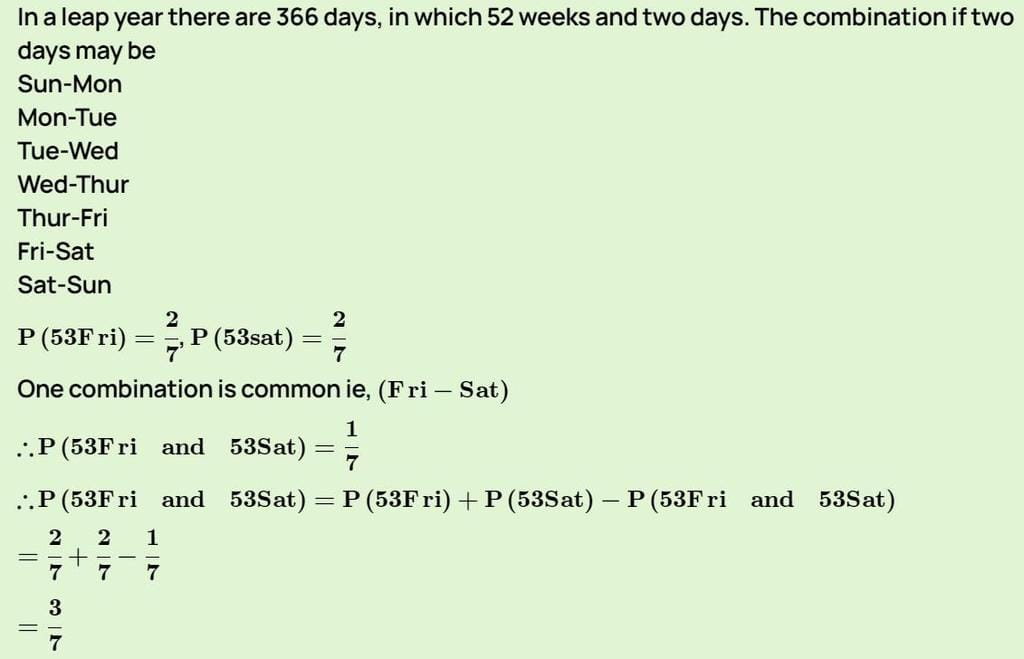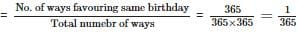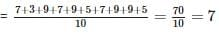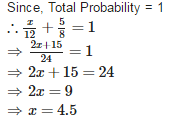RD Sharma Test: Probability

# RD Sharma Test: Probability

Test Description

## 25 Questions MCQ Test Mathematics (Maths) Class 10 | RD Sharma Test: Probability

RD Sharma Test: Probability for Class 10 2023 is part of Mathematics (Maths) Class 10 preparation. The RD Sharma Test: Probability questions and answers have been prepared according to the Class 10 exam syllabus.The RD Sharma Test: Probability MCQs are made for Class 10 2023 Exam. Find important definitions, questions, notes, meanings, examples, exercises, MCQs and online tests for RD Sharma Test: Probability below.
Solutions of RD Sharma Test: Probability questions in English are available as part of our Mathematics (Maths) Class 10 for Class 10 & RD Sharma Test: Probability solutions in Hindi for Mathematics (Maths) Class 10 course. Download more important topics, notes, lectures and mock test series for Class 10 Exam by signing up for free. Attempt RD Sharma Test: Probability | 25 questions in 25 minutes | Mock test for Class 10 preparation | Free important questions MCQ to study Mathematics (Maths) Class 10 for Class 10 Exam | Download free PDF with solutions
 1 Crore+ students have signed up on EduRev. Have you?
RD Sharma Test: Probability - Question 1

### An unbiased die is thrown once. The probability of getting a prime number is

Detailed Solution for RD Sharma Test: Probability - Question 1

Number of possible outcomes = {2, 3, 5} = 3
Number of Total outcomes = 6
∴ Probability of getting a prime number = 3/6 = 1/2

RD Sharma Test: Probability - Question 2

### The probability that a non leap year will have 53 Fridays and 53 Saturdays is

Detailed Solution for RD Sharma Test: Probability - Question 2RD Sharma Test: Probability - Question 3

### A number is selected at random from 1 to 75. The probability that it is a perfect square is

Detailed Solution for RD Sharma Test: Probability - Question 3

Number of possible outcomes = {1, 4, 9, 16, 25, 36, 49, 64} = 8
Number of Total outcomes = 75
∴ Probability of getting a perfect square = 8/75

RD Sharma Test: Probability - Question 4

Ram and Shyam are friends. The probability that both will have the same birth day is

Detailed Solution for RD Sharma Test: Probability - Question 4

Ignoring a leap year, both Ram and Shyam can have any day of the year as their birthday.
∴ Total number of possible ways in which their birthday can fall =
Also they can share the same birthday falls on same date = 365
∴ Required ProbabilityRD Sharma Test: Probability - Question 5

Which of the following can not be the probability of an event?

Detailed Solution for RD Sharma Test: Probability - Question 5

17/16 cannot be the probability of an event because probability of an event cannot be greater than 1.

RD Sharma Test: Probability - Question 6

A die is thrown once. The probability of getting an even number and a multiple of 3 is

Detailed Solution for RD Sharma Test: Probability - Question 6

Number of possible outcomes = {6} = 1
Number of Total outcomes = 6
∴ Required Probability = 1/6

RD Sharma Test: Probability - Question 7

The probability of selecting a queen of diamonds when a card is drawn from well shuffled pack of 52 cards is

Detailed Solution for RD Sharma Test: Probability - Question 7

Number of possible outcomes (queen of diamonds) = 1
Number of Total outcomes = 52
Required Probability = 1/52

RD Sharma Test: Probability - Question 8

A piggy bank contains 100 fifty paise coins, 50 one rupee coins, 20 two rupee coins and 10 five rupee coins. One coin is drawn at random. The probability that the coin drawn will not be a five rupee coin is

Detailed Solution for RD Sharma Test: Probability - Question 8

Number of total coins = 100 + 50 + 20 + 10 = 180
Number of coins except five rupee coins = 180 – 10 = 170
∴ Required Probability = 170/180 = 17/18

RD Sharma Test: Probability - Question 9

A letter of English alphabets is chosen at random. The probability that the letter is consonant

Detailed Solution for RD Sharma Test: Probability - Question 9

Number of consonants = 21
Number of total outcomes = 26
∴ Required Probability = 21/26

RD Sharma Test: Probability - Question 10

If the probability of an event is ‘p’, the probability of its complementary event will be

Detailed Solution for RD Sharma Test: Probability - Question 10

If the probability of an event is pp, the probability of its complementary event will be 1−p.

RD Sharma Test: Probability - Question 11

When a die is thrown, the probability of getting an odd number less than 3 is

Detailed Solution for RD Sharma Test: Probability - Question 11

Number of possible outcomes = {1} = 1
Number of Total outcomes = 6
∴ Required Probability = 1/6

RD Sharma Test: Probability - Question 12

A card is drawn from a pack of 52 cards at random. The probability of getting neither an ace nor a king card is

Detailed Solution for RD Sharma Test: Probability - Question 12

Number of Total outcomes = 52
Number of aces and Number of kings = 4 + 4 = 8
Number of cards except ace and king = 52 – 8 = 44
Required Probability = 44/52 = 11/13

RD Sharma Test: Probability - Question 13

The probability that a non leap year selected at random will have 53 Sundays is

Detailed Solution for RD Sharma Test: Probability - Question 13

Non-leap year contains 365 days = 52 weeks + 1 days
52 weeks contain 52 Sundays
We will get 53 Sundays if one Sunday will come in a week.
Number of Total possible outcomes = 7
Number of possible outcomes = 1
∴ Required Probability = 1/7

RD Sharma Test: Probability - Question 14

Cards marked with numbers 1, 2, 3, ……….., 25 are placed in a box and mixed thoroughly and one card is drawn at random from the box. The probability that the number on the card is a multiple of 3 or 5 is

Detailed Solution for RD Sharma Test: Probability - Question 14

Number of multiples of 3 = 8
Number of multiples of 5 = 5
Number of possible outcomes = 8 + 5 = 13
Number of Total outcomes = 25
∴ Required Probability = 13/25

RD Sharma Test: Probability - Question 15

A child’s game has 8 triangles of which 5 are blue and rest are red and 10 squares of which 6 are blue and the rest are red. One piece is lost at random. The probability that it is a square of blue colour is

Detailed Solution for RD Sharma Test: Probability - Question 15

Number of possible outcomes = 6
Number of total outcomes = 8 + 10 = 18
∴ Required Probability = 6/18 = 1/3

RD Sharma Test: Probability - Question 16

A number is selected at random from the numbers 7, 3, 9, 7, 9, 5, 7, 9, 9, 5. The probability that the selected number is their average is

Detailed Solution for RD Sharma Test: Probability - Question 16

Average of given numbersNumber of outcomes = 3
Number of total outcomes = 10
∴ Required Probability = 3/10

RD Sharma Test: Probability - Question 17

A card is drawn from a pack of 52 cards at random. The probability of getting either an ace or a king card is

Detailed Solution for RD Sharma Test: Probability - Question 17

Number of Total outcomes = 52
Number of aces and Number of kings = 4 + 4 = 8
Required Probability = 8/52 = 2/13

RD Sharma Test: Probability - Question 18

There are 20 boys and 15 girls in a class. A student is chosen as leader at random. The probability that the leader is a boy is

Detailed Solution for RD Sharma Test: Probability - Question 18

Number of possible outcomes = 20
Number of Total outcomes = 20 + 15 = 35
∴ Required Probability = 20/35 = 4/7

RD Sharma Test: Probability - Question 19

A letter of English alphabets is chosen at random. The probability that the letter chosen is a vowel is

Detailed Solution for RD Sharma Test: Probability - Question 19

Number of consonants = 5
Number of total outcomes = 26
∴ Required Probability = 5/26

RD Sharma Test: Probability - Question 20

A bag contains 50 balls of which 2x are red, 3x are white and 5x are blue. A ball is selected at random. The probability that it is not white is

Detailed Solution for RD Sharma Test: Probability - Question 20

Here
2x + 3x + 5x = 50 = ⇒ 10x = 50 ⇒ x = 5
Number of red balls = 2 x 5 = 10
Number of white balls = 3 x 5 = 15
Number of blue balls = 5 x 5 = 25
Now, Number of possible outcomes = 25 + 10 = 35
And Number of total outcomes = 50
∴ Required Probability = 35/50 = 7/10

RD Sharma Test: Probability - Question 21

A box contains 3 blue balls, 2 white balls and 4 red balls. If a ball is drawn at random from the box, the probability of getting a white ball is

Detailed Solution for RD Sharma Test: Probability - Question 21

Number of outcomes = 2
Number of total outcomes = 3 + 2 + 4 = 9
∴ Required Probability = 2/9

RD Sharma Test: Probability - Question 22

The probability of a sure event is

Detailed Solution for RD Sharma Test: Probability - Question 22

The probability of a sure event is always 1.

RD Sharma Test: Probability - Question 23

From a well shuffled pack of 52 cards, one card is drawn at random. The probability of getting a black king is

Detailed Solution for RD Sharma Test: Probability - Question 23

Number of possible outcomes = 2
Number of Total outcomes = 52
∴ Required Probability = 2/52 = 1/26

RD Sharma Test: Probability - Question 24

Cards marked with numbers 1, 2, 3, ……….., 25 are placed in a box and mixed thoroughly and one card is drawn at random from the box. The probability that the number on the card is a multiple of 3 and 5 is

Detailed Solution for RD Sharma Test: Probability - Question 24

Explanation : Multiple of 3 : 3,6,9,12,15,18,21,24

Multiple of 5 : 5,10,15,20,25

Number of possible outcomes (multiple of 3 and 5) = {15} = 1

Number of Total outcomes = 25

∴ Required Probability = 1/25

RD Sharma Test: Probability - Question 25

The probability of guessing the correct answer to certain text questions is x / 12. If the probability of not guessing the answer is 5/8, then the value of x is

Detailed Solution for RD Sharma Test: Probability - Question 25## Mathematics (Maths) Class 10

53 videos|403 docs|138 tests
 Use Code STAYHOME200 and get INR 200 additional OFF Use Coupon Code
Information about RD Sharma Test: Probability Page
In this test you can find the Exam questions for RD Sharma Test: Probability solved & explained in the simplest way possible. Besides giving Questions and answers for RD Sharma Test: Probability, EduRev gives you an ample number of Online tests for practice

## Mathematics (Maths) Class 10

53 videos|403 docs|138 tests